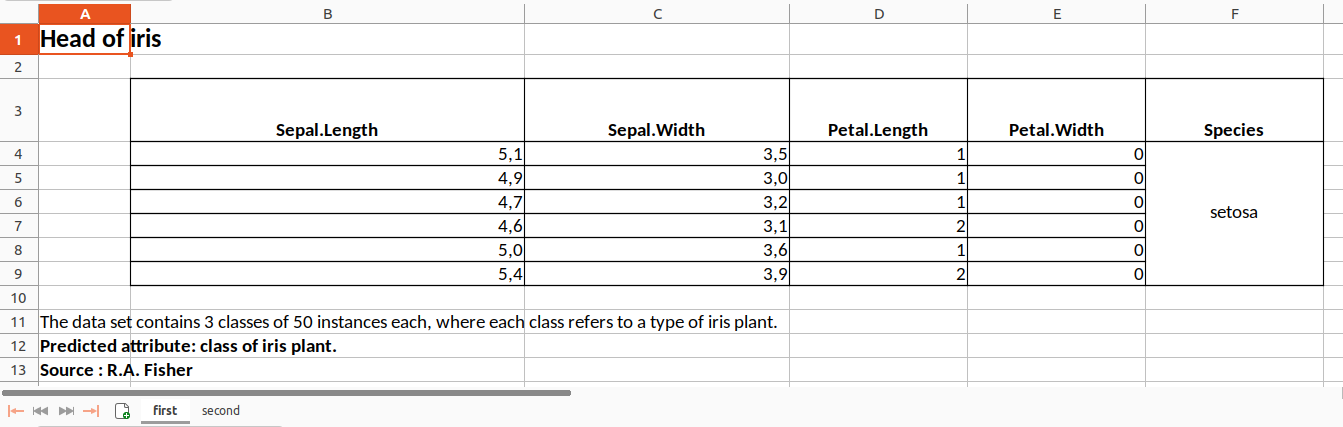library(tablexlsx)
library(dplyr, warn.conflicts = FALSE)

Let’s define an export folder that will be the same for all the examples on this page

mypath <- tempdir()

Important : in this vignette, all examples use %>% to pass the object argument in the toxlsx() function, but it works interchangeably with these 3 syntaxes below :

toxlsx(object = iris, path = mypath)
iris |> toxlsx(path = mypath)
iris %>% toxlsx(path = mypath)

### 1. Simply export a data frame to an xlsx file

iris %>% toxlsx(path = mypath)
#> ✔ Your Excel file 'Export.xlsx' is available in the folder '/tmp/RtmpI1LtqY'

Preview of the xlsx file in LibreOffice Calc :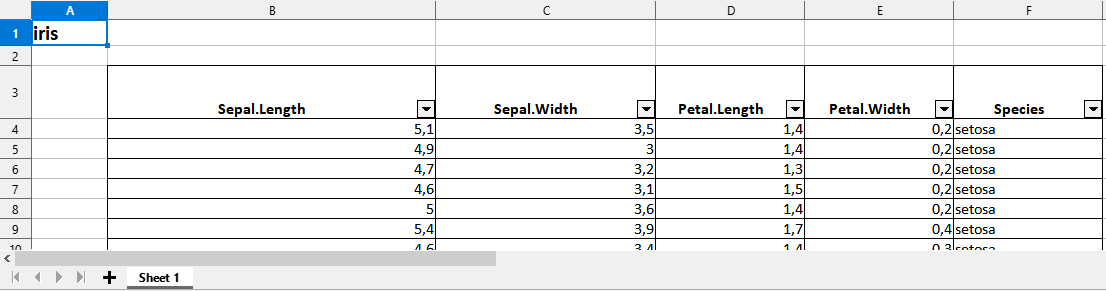### 2. Export a list of several data frames to an xlsx file (each data frame in a different sheet)

list(iris,cars) %>% toxlsx(path = mypath)
#> ✔ Your Excel file 'Export.xlsx' is available in the folder '/tmp/RtmpI1LtqY'

Preview of the xlsx file in LibreOffice Calc :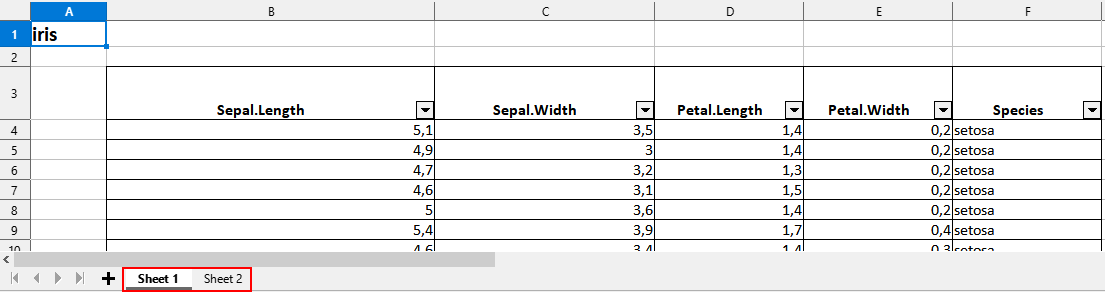### 3. Export a data frame to an xlsx file (merging modalities for one column)

# Create df1
df1 <- data.frame(
group = c("dupont","dupont","arnold","arnold"),
name = c("toto","tata","tutu","tete"),
volume = c(10,8,12,15)
)
#  Export
df1 %>% toxlsx(path = mypath, mergecol = "group")
#> ✔ Your Excel file 'Export.xlsx' is available in the folder '/tmp/RtmpI1LtqY'

Preview of the xlsx file in LibreOffice Calc :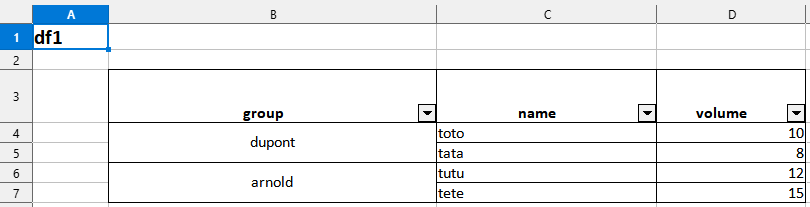### 4. Export a data frame to an xlsx file (merging modalities for several columns)

# Create df2
df2 <- data.frame(
country = c(rep("france",4),rep("england",4)),
group = c(rep("dupont",2),rep("martin",2),
rep("arnold",2),rep("harry",2)),
name = c("toto","tata","tutu","tete",
"momo","mama","mumu","meme"),
volume = c(10,8,12,15,
14,10,5,12)
)
#  Export
df2 %>% toxlsx(path = mypath, mergecol = c("country","group"))
#> ✔ Your Excel file 'Export.xlsx' is available in the folder '/tmp/RtmpI1LtqY'

Preview of the xlsx file in LibreOffice Calc :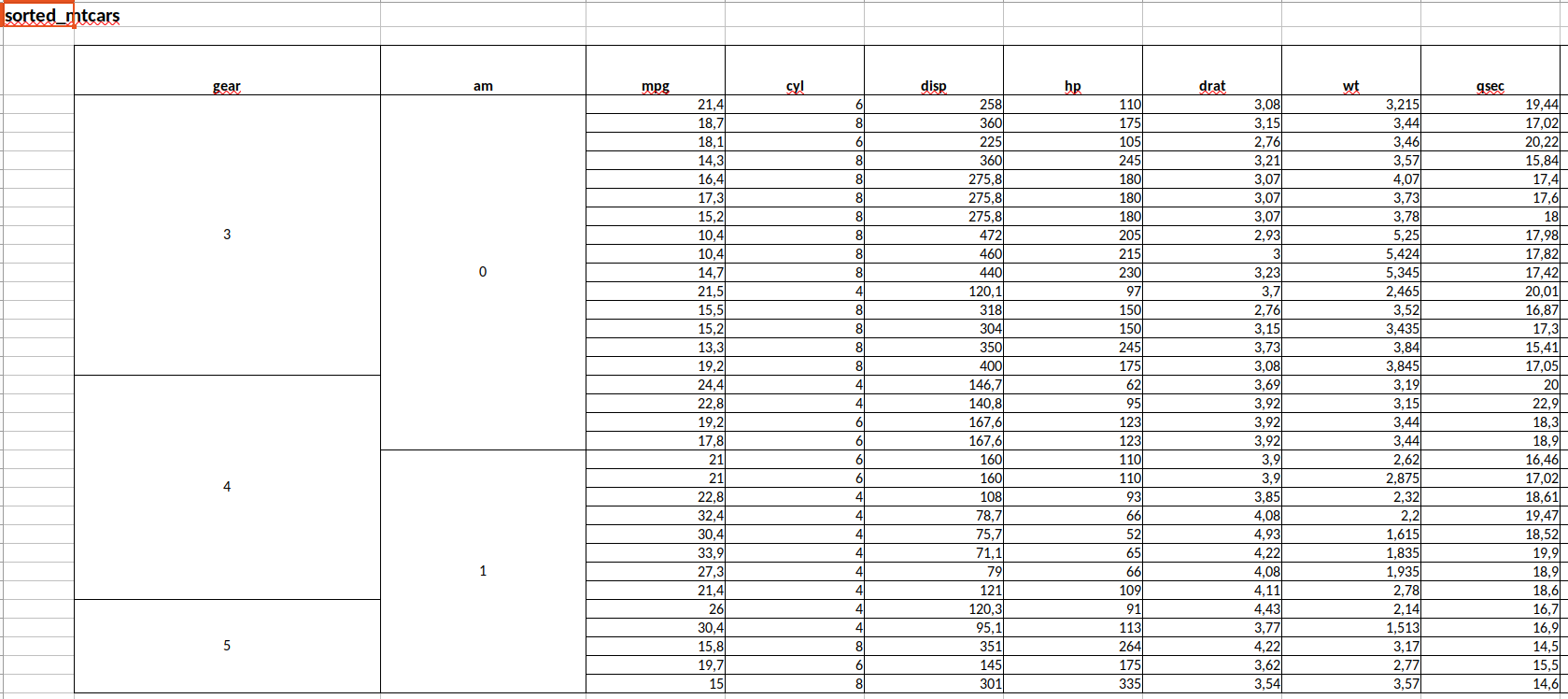### 5. Export a list of several data frames to an xlsx file (several data frames in a same sheet)

tb1 <- data.frame(tables = c(rep("iris",5),rep("cars",2)),
var = c(names(iris),names(cars)))

tb2 <- data.frame(tables = c("iris","cars","cars"),
rownumber = c(150,50,32))

list(tb1,tb2) %>%
toxlsx(tosheet = list("tb1" = "mydata",
"tb2" = "mydata"),
footnote1 = list("tb1" = "The data set contains 3 classes of 50 instances each, where each class refers to a type of iris plant.",
"tb2" = "The data give the speed of cars and the distances taken to stop. Note that the data were recorded in the 1920s."),
footnote2 = list("tb1" = "Predicted attribute: class of iris plant.",
"tb2" = "Data recorded in the 1920s"),
footnote3 = list("tb1" = "Source : R.A. Fisher",
"tb2" = "Source : M. Ezekiel"),
path=mypath)
#> ✔ Your Excel file 'Export.xlsx' is available in the folder '/tmp/RtmpI1LtqY'

Preview of the xlsx file in LibreOffice Calc :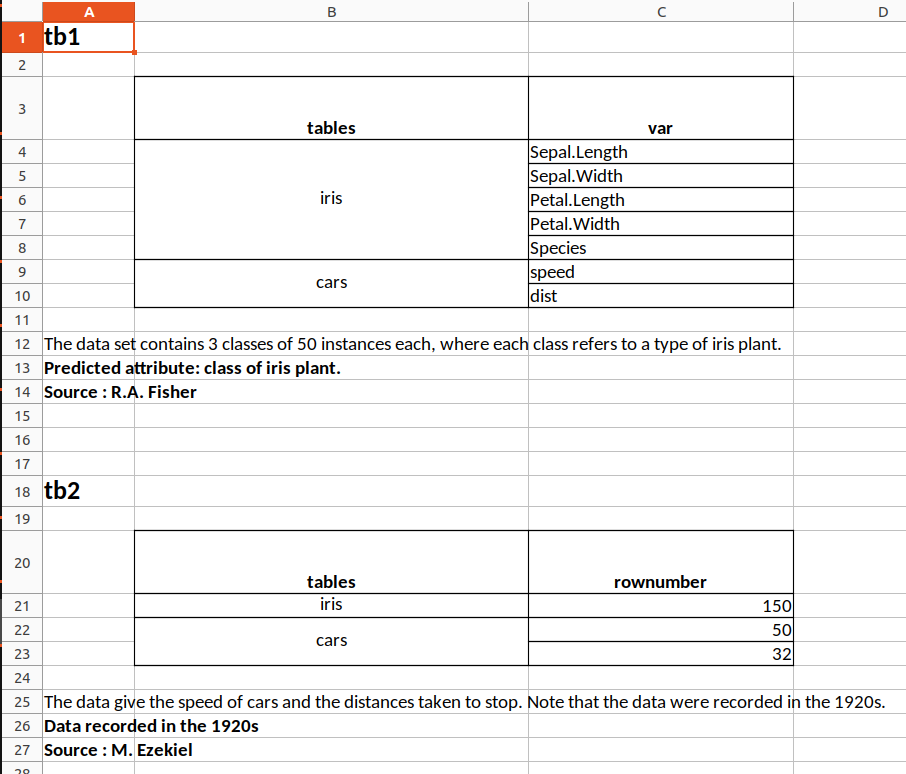### 6. Export a list of data frames to an named xlsx file by specifying which data frame goes in which sheet, styling each column, giving a title and footnotes…

iris <- iris %>% head()

list(iris,cars) %>%
toxlsx(tosheet = list("iris" = "first",
"cars" = "second"),
title = list("iris" = "Head of iris",
# The columnstyle argument is optional in toxlsx().
# It is used only if you want to specify the format of each column
columnstyle = list("iris" = list("c1" = "decimal",
"c2" = "decimal",
"c3" = "number",
"c4" = "number",
"c5" = "character"),
"cars" =  list("c1" = "number",
"c2" = "number")),
footnote1 = list("iris" = "The data set contains 3 classes of 50 instances each, where each class refers to a type of iris plant.",
"cars" = "The data give the speed of cars and the distances taken to stop. Note that the data were recorded in the 1920s."),
footnote2 = list("iris" = "Predicted attribute: class of iris plant.",
"cars" = "Data recorded in the 1920s"),
footnote3 = list("iris" = "Source : R.A. Fisher",
"cars" = "Source : M. Ezekiel"),
filename = "Results",
path = mypath)
#> ✔ Your Excel file 'Results.xlsx' is available in the folder '/tmp/RtmpI1LtqY'

The equivalent with the {openxlsx} syntax would be much longer and more painful to write. See here for the equivalent of the sixth example.

Preview of the xlsx file in LibreOffice Calc :